Next: Convective Time Derivative Up: Mathematical Models of Fluid Previous: Conservation Laws

# Mass Conservation

Let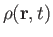andbe the mass density and velocity of a given fluid at point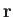and time. Consider a fixed volume, surrounded by a surface. The net mass contained withinis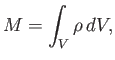(1.32)

where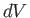is an element of. Furthermore, the mass flux across, and out of, is [see Equation (1.29)]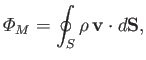(1.33)

whereis an outward directed element of. Mass conservation requires that the rate of increase of the mass contained within, plus the net mass flux out of, should equal zero: that is,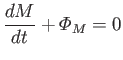(1.34)

[cf., Equation (1.31)]. Here, we are assuming that there is no mass generation (or destruction) within(because individual molecules are effectively indestructible). It follows that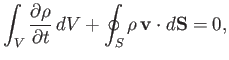(1.35)

becauseis non-time-varying. Making use of the divergence theorem (see Section A.20), the previous equation becomes(1.36)

However, this result is true irrespective of the size, shape, or location of volume, which is only possible if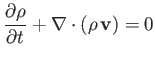(1.37)

throughout the fluid. The previous expression is known as the equation of fluid continuity, and is a direct consequence of mass conservation.Next: Convective Time Derivative Up: Mathematical Models of Fluid Previous: Conservation Laws
Richard Fitzpatrick 2016-03-31